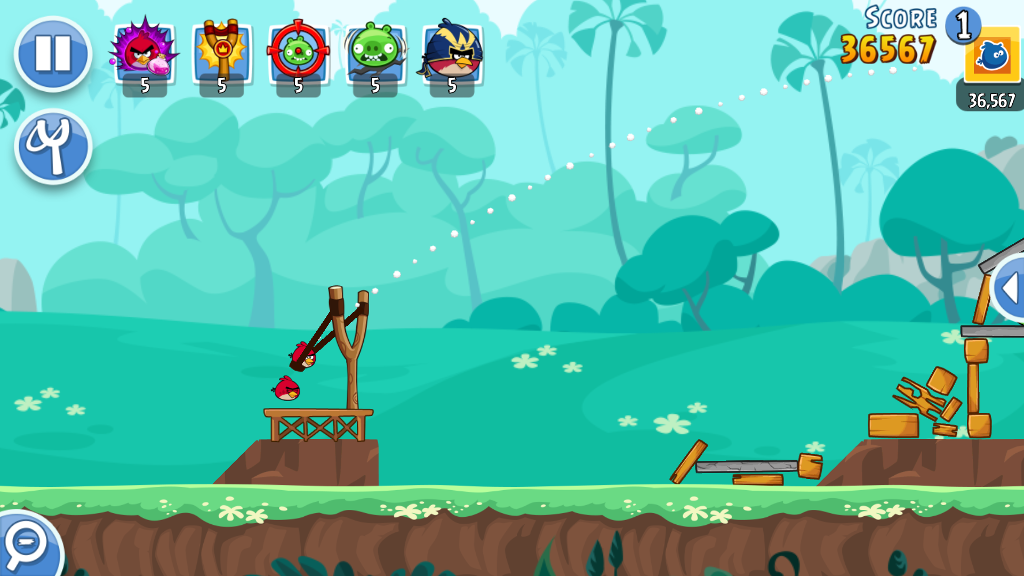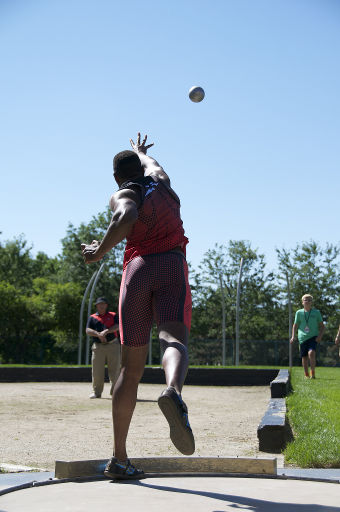# Trajectory of a projectile: the Angry Birds problem

Differential equations are not only used to predict when you will arrive somewhere.

Like at least 200m other people, you may have run into this issue at one point of your life.How far should you drag the little bird to slay the evil pigs?

First, let us make an observation. However you throw the bird, it always flies according to a parabolic trajectory.

This is because the game is inspired by realphysics. The trajectory of real projectiles flung into the air depend on two things:

• Its speed as it becomes airborne (in Angry Birds, this depends on how far back you draw the bird, and in which direction)
• The forces exerted on it: assuming we neglect friction, the only force exerted on the flying projectile is gravity (its weight). We could write differential equations using Newton’s 2nd law to get an exact result, but let us keep this intuitive first.

If we fling the bird upwards and forwards, then we can decompose its initial speed as it is released into two components: an horizontal component and a vertical component.

As you probably know, weight (the only force exerted on the bird once it is released) is directed vertically, downwards. Therefore, it has no horizontal component.

So what is going to happen next?

• On the horizontal axis, the bird is going to keep its horizontal velocity because no force affects it.
• On the vertical axis though, we have opposite tendencies. Because of its initial velocity, the bird is rising upwards. However, its weight is pulling it down, so it progressively slows down until it comes to a stop in the vertical axis. Then its starts falling down, faster and faster. If you try to simulate the motion of the bird by drawing with a pencil on a piece of paper, you should get something that looks like a parabola.

Now, if you want to go further, we are going to need these differential equations. The weight of the projectile is the product of its mass $m$) and the gravitational acceleration $g$, so according to Newton’s Second Law:

$$m\frac{d^2x}{dt^2}=0$$ $$m\frac{d^2y}{dt^2}=-mg$$

We integrate twice to get the trajectory of the projectile.

$$\frac{dx}{dt}=v_x$$ $$\frac{dy}{dt}=v_y-mgt$$

$$x(t)=v_x.t$$ $$y(t)=v_y.t-\frac{1}{2}gt^2$$

We can then substitute $x$ in the equation of $y$.

$$y(t)=\frac{v_y}{v_x}x(t)-\frac{1}{2}\frac{g}{{v_x}^2}x(t)^2$$

We want to know at what distance ($x$) the projectile hits the ground ($y=0$). This requires solving a second degree equation, which yields to solutions.

$$y(t)=0\Leftrightarrow x(t)=0 \quad or \quad x(t)=\frac{2}{g}v_x v_y$$

We discard the first as it is the launching point. The second one gives an equation for the range of the projectile depending on its launching speed (and the gravitational acceleration).

We can go further and study which angle to shoot at to reach the maximal range. To do this, we first substitute $v_y$ in the equation.

$$v_x^2+v_y^2=v^2 \Rightarrow v_y=\sqrt{v^2-v_x^2} \ x(t)=\frac{2}{g}v_x \sqrt{v^2-v_x^2}$$

We then differentiate the equation of $x$ with respect to $v_x$.

$$\frac{dx(t)}{dv_x}=\frac{2}{g}\left( \sqrt{v^2-v_x^2}-v_x^2\frac{1}{\sqrt{v^2-v_x^2}}\right )$$

The maximum range is reached when the derivative is 0.

$$\frac{dx(t)}{dv_x}=0\Rightarrow v_x^2=v^2-v_x^2$$

We recognize the expression of $v_y$ there.

$$v_x^2=v_y^2$$

As both $v_x$ and $v_y$ are positive:

$$v_x=v_y$$

The optimal angle to launch a projectile and reach the maximal range is therefore 45 degrees.Photo by Koji KawanoThis result does not only apply to Angry Birds, but also to real sports, like the shot put, in which the optimal throwing angle is also close to 45 degrees.

Two putting styles are in current general use by shot put competitors: the _glide_ and the spin. With all putting styles, the goal is to release the shot with maximum forward velocity at an angle of approximately forty degrees. (Source: Wikipedia)

Banner photo by Nick Harris1Share